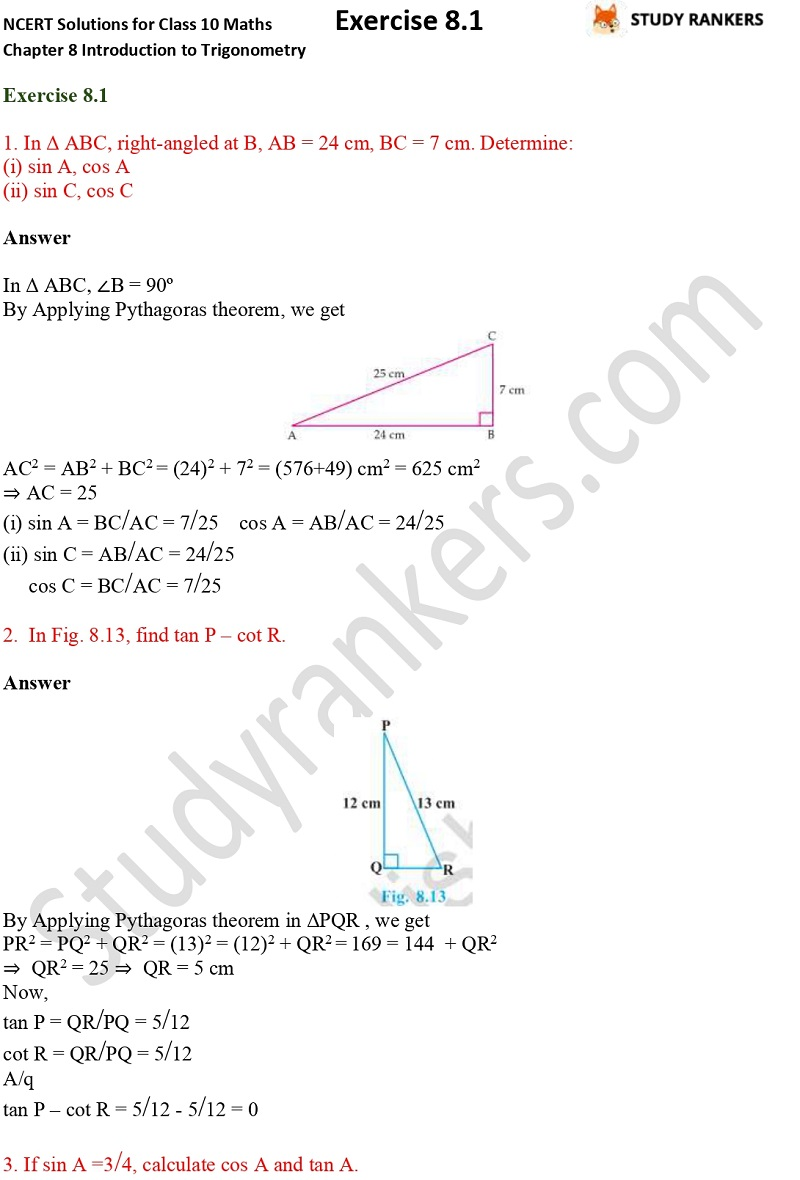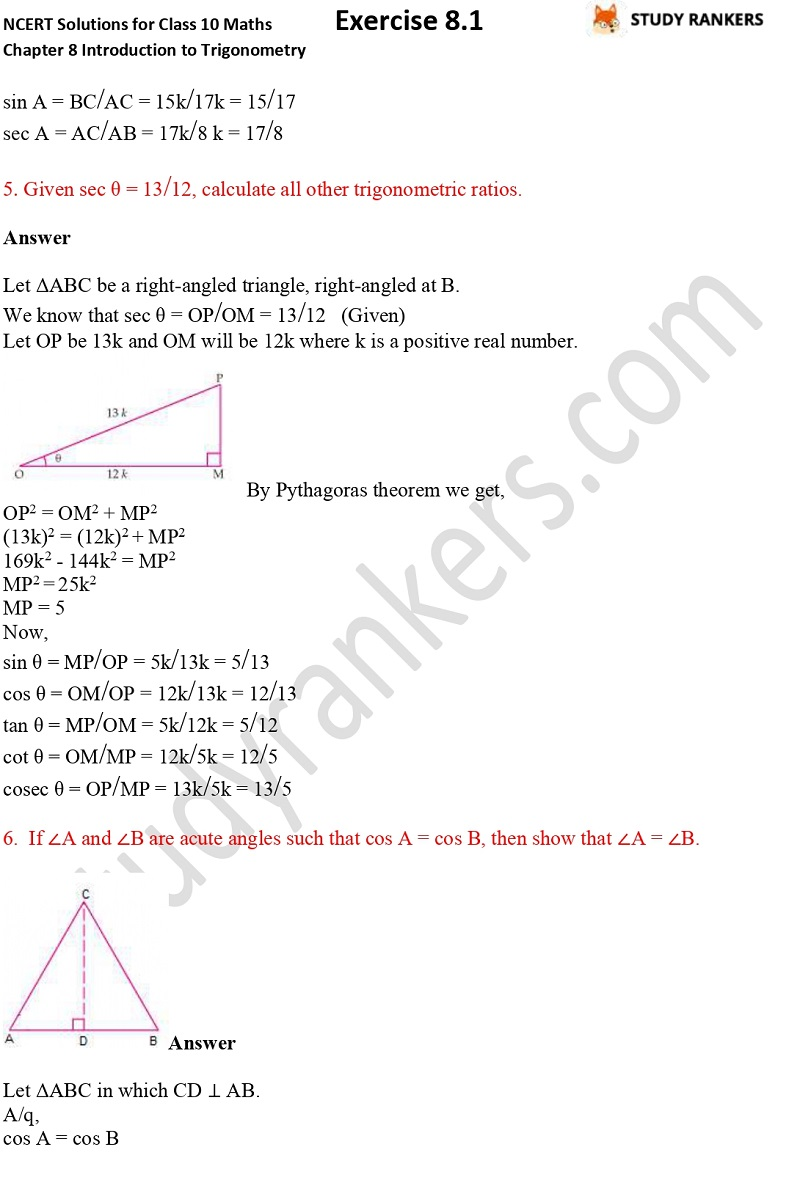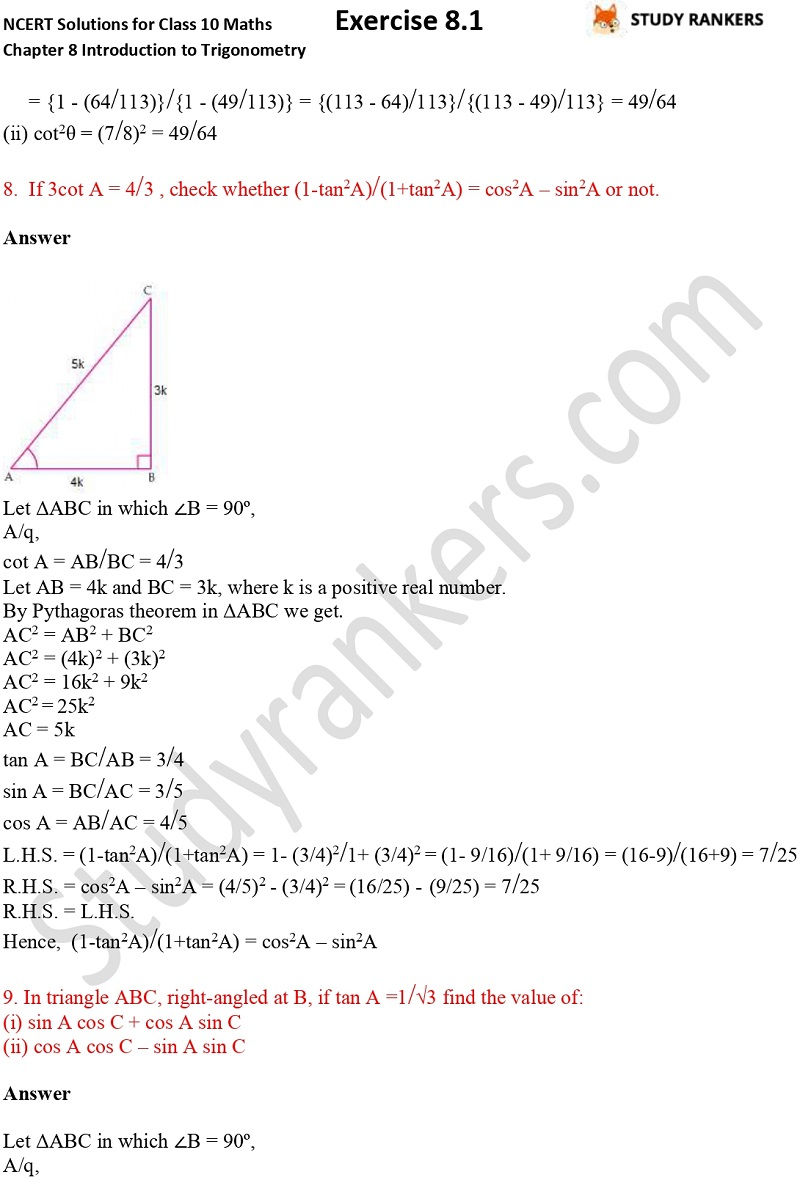## NCERT Solutions for Class 10 Maths Chapter 8 Introduction To Trigonometry Exercise 8.1

Chapter 8 Introduction To Trigonometry Exercise 8.1 Math Class 10 Solutions is provided here which is developed according to the latest marking scheme released by CBSE. You can use these CBSE NCERT Solutions to check your answers and prepare better answers to secure good marks in board exams of Class 10. It will also help you in understanding the basic concepts of the chapters and types of questions that could come in the examinations.

There are total 11 questions in this exercise which has various types of question like  If ∠A and ∠B are acute angles such that cos A = cos B, then show that ∠A = ∠B, Given sec θ = 13/12, calculate all other trigonometric ratios etc.